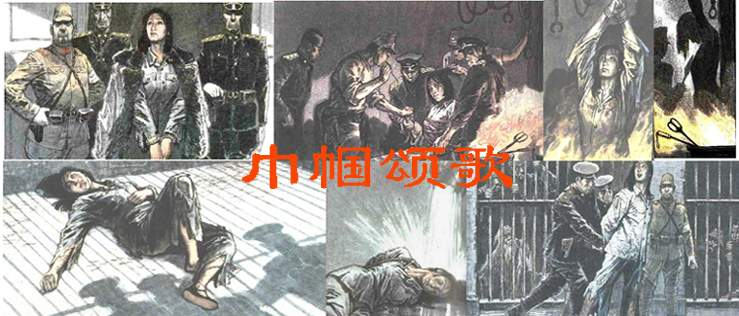^^^^^^^^^^^^^^^^^^^^^^^^^^^^^^^^^^^^^^^^^^^^^^^^^^

^^2005年见证：^^^^^^^^^^^^^^^^^^^^^^^^^^^^^^^^^^^^^^^^^^^^^^^^
[抗日战争胜利60周年，赵一曼烈士诞辰100周年]专题

配合社会大规模读物、影视等媒体宣传活动，希望将您真挚的话语留下（请您留言|人物讨论 ），共同重温历史，缅怀烈士！！！

^^^^^^^^^^^^^^^^^^^^^^^^^^^^^^^^^^^^^^^^^^^^^^^^^^^^